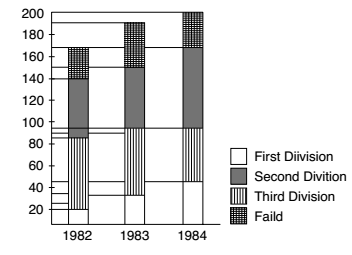## Bar graph

#### Data Interpretation

Direction: The following bar graph depicts the result for BSc students of a college for 3 years. Read the graph and answer the questions based on this graph.1. The percentage of students passed in first division in 1982 was

1.  The percentage of students = Total passed students in first division in 1982 x 100 Total number of students in 1982

##### Correct Option: D

As per given Bar graph we can calculate that,
Total passed students in first division in 1982 = 20
Total number of students in 1982 = 170

 The percentage of students = Total passed students in first division in 1982 x 100 Total number of students in 1982

 The percentage of students = 20 x 100 170
 The percentage of students = 200 = 11 13 % 17 17

1. The percentage of students failed in 1984 was

1. ∴ Percentage of students failed in 1984

 = total number of failed students in 1984 × 100 % total number of students in 1984

##### Correct Option: C

∴ Percentage of students failed in 1984

 = total number of failed students in 1984 × 100 % total number of students in 1984

Percentage of students failed in 1984
 = 35 × 100 = 17 1 % 200 2

1. The percentage of students passed in 1982 was

1.  The percentage of students passed in 1982 = Total passed students in 1982 x 100 Total number of students in 1982

##### Correct Option: D

As per given Bar graph we can see that,
Total passed students in 1982 = 140
Total number of students in 1982 = 170

 The percentage of students passed in 1982 = Total passed students in 1982 x 100 Total number of students in 1982
 Required percentage = 140 x 100 170

 = 1400 = 82 6 % 17 17

1. The aggregate pass percentage during the 3 years was

1. As per given Bar graph we can see that,
Total passed students = 140 + 150 + 165 = 455
Total students = 170 + 195 + 200 = 565

 ∴ Required percentage = Total passed students x 100 Total students

##### Correct Option: C

As per given Bar graph we can see that,
Total passed students = 140 + 150 + 165 = 455
Total students = 170 + 195 + 200 = 565

 ∴ Required percentage = Total passed students x 100 Total students

 = 455 x 100 565

 = 9110 = 80 60 % 113 113

1. The number of students passed in third division in 1984 was

1. Required number of students passed in third division = Total number of passed students in 1984 years − Total number of students passed in first and second division in 1984 years

##### Correct Option: C

Required number of students passed in third division = Total number of passed students in 1984 years − Total number of students passed in first and second division in 1984 years
Required number of students passed in third division = 165 − 95 = 70